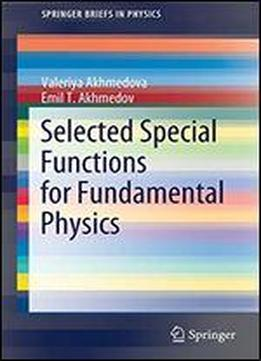# Selected Special Functions For Fundamental Physics (springerbriefs In Physics) by Valeriya Akhmedova / 2020 / English / PDF

This book presents calculation methods that are used in both mathematical and theoretical physics. These methods will allow readers to work with selected special functions and more generally with differential equations, which are the most frequently used in quantum mechanics, theory of relativity and quantum field theory. The authors explain various approximation methods used to solve differential equations and to estimate integrals. They also address the basics of the relations between differential equations, special functions and representation theory of some of the simplest algebras on the one hand, and fundamental physics on the other. Based on a seminar for graduate physics students, the book offers a compact and quick way to learn about special functions. To gain the most from it, readers should be familiar with the basics of calculus, linear algebra, and complex analysis, as well as the basic methods used to solve differential equations and calculate integrals.

views: 401